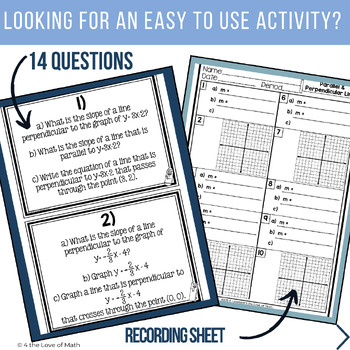Parallel and Perpendicular Lines: Carousel ActivitySubject
Resource Type
Common Core State Standards
Product Rating
File Type

PDF (Acrobat) Document File

Be sure that you have an application to open this file type before downloading and/or purchasing.

4 MB|7 pages
Share
Product Description

Included in this parallel line and perpendicular line activity game are 5 pages of questions, a student recording sheet, and an answer key. The assignment has students determine the slope of lines parallel or perpendicular to other lines.

There are 10 questions included, 4 different types of questions. All equations are in slope intercept form.

-3 questions that ask students to determine the slope of a line parallel and perpendicular to the graph of an equation, as well as the equation of a line that is parallel to the graph of an equation & passes through a certain point.

-3 questions that ask students to determine the slope of a line parallel & perpendicular to the graph of an equation, as well as the equation of a line that is perpendicular to the graph of an equation & passes through a certain point.

-2 question cards that ask students to find the slope of a line parallel to the graph of an equation, to graph the equation, then to graph a line parallel to the graph that passes through a certain point.

-2 question cards ask students to find the slope of a line perpendicular to the graph of an equation, to graph the equation, then to graph a line perpendicular to the graph that passes through a certain point.

To play:

Cut each question page in half to separate the question boxes.

Hang the 10 questions around the classroom.

Make enough copies of the recording sheets for each student or each group of students.

Divide students up into groups of 2-3 students. Assign each group a starting number. (Ex. Group 1 may start at problem 1, group 2 at problem 2, etc.) Each group of students will begin at their assigned spot. They will work together to solve the problem on the card, and record their answers on the recording sheet. Set a timer for 3-4 min. Once the timer rings students will rotate (like a carousel) and solve the next problem. Students will continue rotating until they have completed each problem.

To complete this activity students need to know how to find the slope of a line that is parallel or perpendicular to the graph of a given equation. They also must be able to graph a line using slope intercept form, as well as graphing a line using the slope of a line and a point on the line. Finally, they must be able to find the equation of a line given a point the line crosses through and using their knowledge of parallel & perpendicular lines.

****This activity is a part of my “Algebra Carousel Activity Bundle” which can be found here: Carousel Activity Bundle

If you have any questions, comments, suggestions, etc, please email me at: 4theLoveofMath2@gmail.com

Thank you!

*****************************************************************************

This item is a paid digital download. As such, it is for use in one classroom (or by one teacher) only. This item is bound by copyright laws and redistributing, editing, selling, or posting this item (or any part thereof) on the Internet are all strictly prohibited without first gaining permission from the author. Violations are subject to the penalties of the Digital Millennium Copyright Act.

*****************************************************************************

Total Pages
7 pages
N/A
Teaching Duration
N/A
Report this Resource to TpT
Reported resources will be reviewed by our team. Report this resource to let us know if this resource violates TpT’s content guidelines.